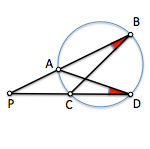# Metric geometry : Generalization of the concept of “Power”The power concept of a point of a circumference product is based on the most for the least of the distances of a point to a circle.

These distance values ​​are given in the string that contains the center of the circle and the point, namely, in diameter containing said point.

Is it possible to generalize this concept to consider other strings that pass through the point P?

Power W to a point P on a circumference c product is the most for the least distance from the point P the circumference c.

If we consider the straight lines passing through a point P and severed at a circumference c, cut points with said circumference (A, B, C and D) determine two similar triangles:

• PCB

Indeed, to apply the concepts of arc capable of a segment, we see that the angles B and D must be equal to be registered in the circle passing through the four point. Furthermore triangles share the vertex P and hence the angle, and accordingly are similar.

Applying Thales theorem the two similar triangles have to:

PA / PD = PC / PB

and therefore

PA * PB = PC * PD = Constant

This shows that Power from the point P is independent of the chosen line, as we wanted to prove.

Metric geometry